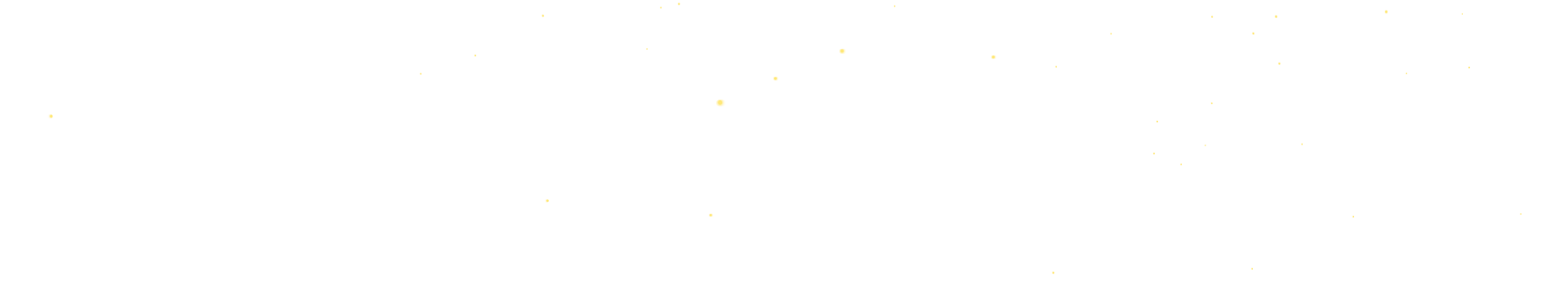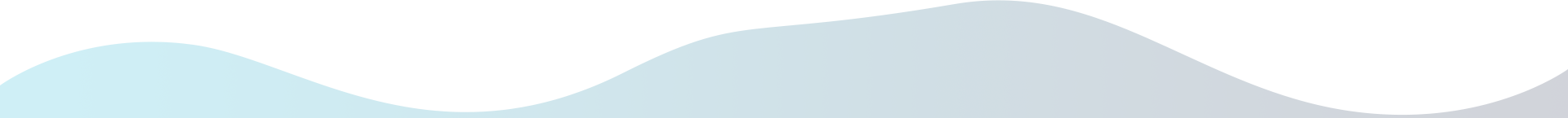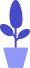# Python turtle module combat: drawing body of the robot cat

Estimated reading: 7 minutes 64 views

We have drawn the head of the robot cat in the previous tutorial, and in this tutorial we will draw the backbone of the robot cat.

## body() function

Next we define the body() function, which is used to draw the robot cat’s body. The function first draws a blue rectangle to represent the body, and then draws a white circle to represent the robot cat’s belly. Next, draw a red rectangle representing the red ribbon that separates the head from the body. Finally, draw a semi-circle to represent the robot cat’s legs.

The code for the body() function is shown below.

``````def body():
#blue body
color("blue","blue")
penup()
goto(-50,-40)
drawRect(100,80,True)
#white belly
color("white","white")
penup()
goto(0,-30)
drawRound(40,True)
#red ribbon
color("red", "red")
penup()
goto(-60,-35)
drawRect(120,10,True)
#white legs
color("white","white")
penup()
goto(15,-127)
pendown()
begin_fill()
circle(14,180)
end_fill()``````

First call the color() function to set both the brush and fill colors to blue. Then call the penup() function to lift the brush without leaving handwriting on the canvas. Call the goto() function to move the pen to the specified position. Then call the custom function drawRect() to draw a rectangle with a length of 100 pixels and a width of 80 pixels, filled with blue, to represent the body of the robot cat.

Then call the color() function to set both the brush and fill colors to white. Then call the penup() function to lift the brush without leaving handwriting on the canvas. Call the goto() function to move the pen to the specified position. Then call the custom function drawRound() to draw a circle with a radius of 40 pixels and filled with white, representing the big belly of the robot cat.

Next, call the color() function again to set both the brush and fill colors to red. Then call the penup() function to lift the brush without leaving handwriting on the canvas. Call the goto() function to move the pen to the specified position. Then call the custom function drawRect() to draw a flat rectangle with a length of 120 pixels and a width of 10 pixels, filled with red, to separate the body and head of the robot. This is the red ribbon for the robot and the place to hang the bell.

Then call the color() function to set both the brush and fill colors to white. Then call the penup() function to lift the brush without leaving handwriting on the canvas. Call the goto() function to move the pen to the specified position. Call the pendown() function to drop the pen. Call setheading(90) to set the direction of movement of the turtle when it starts, that is, to rotate the turtle 90 degrees. Call the begin_fill() function to start filling. Call circle(14,180) to draw a semicircle with a radius of 14 pixels. Then call the end_fill() function to stop filling. This completes the drawing of the two legs of the robot cat.

Call this function to see the drawing effect, as shown in Figure 1.figure 1

## feet() function

Next, we define the feet() function, which is used to draw the robot cat’s feet.

The code for the feet() function is shown below.

```def feet():
#leftfoot
color("black","white")
penup()
goto(-30,-110)
drawRound(20,True)
#rightfoot
color("black","white")
penup()
goto(30,-110)
drawRound(20,True)```

Call the color() function to set the brush color to black and the fill color to white. Then call the penup() function to lift the brush without leaving handwriting on the canvas. Call the goto() function to move the pen to the position where the x coordinate is −30 and the y coordinate is −110. Then call the custom function drawRound() to draw a circle with a radius of 20 pixels and filled with white, representing the left foot of the robot cat.

Then repeat a similar motion, drawing the right foot of the Doraemon. The code is basically the same, but the coordinates of the movement are different, so I won’t repeat them here. Call the feet() function to see the drawing effect, as shown in Figure 2.figure 2

## arms() function

Next, we define the arms() function to draw the arms of the robot cat. Here, two quadrilaterals are used to represent the two arms of the robot cat. The code for the arms() function is shown below.

``````def arms():
#left arm
color("blue","blue")
penup()
begin_fill()
goto(-51,-50)
pendown()
goto(-51,-75)
left(70)
goto(-76,-85)
left(70)
goto(-86,-70)
left(70)
goto(-51,-50)
end_fill()
#right arm
color("blue","blue")
penup()
begin_fill()
goto(49,-50)
pendown()
goto(49,-75)
left(70)
goto(74,-85)
left(70)
goto(84,-70)
left(70)
goto(49,-50)
end_fill()``````

Call the color() function to set both the brush color and fill color to blue. Then call the penup() function to lift the brush without leaving handwriting on the canvas. Call the begin_fill() function to start filling. Call the goto() function to move the pen to the specified position.

Then call the pendown() function to drop the pen. Call the goto() function to draw a straight line. Then call the function left(70), which means to rotate 70 degrees to the left. Call the goto() function to draw the second line. Then call the function left(70), which means to rotate 70 degrees to the left. Call the goto() function to draw the third line. Then call the function left(70), which means to rotate 70 degrees to the left again. Call the goto() function to draw the fourth line.

Call the end_fill() function to complete the color filling. This completes us with a blue filled quad to represent the Doraemon’s left arm.

Then repeat a similar motion, drawing the right arm. The code is basically the same, but the coordinates of the move are different, so I won’t repeat them here. Call the arms() function to see the drawing effect, as shown in Figure 3.image 3

## hands() function

Next, we define the hands() function to draw the robot cat’s hands. Here, two white filled circles are used to represent Doraemon’s hands. The code of the hands() function is similar to that of the feet() function, so I won’t explain too much here, just list the code directly.

``````def hands():
#lefthand
color("black","white")
penup()
goto(-90,-71)
drawRound(15,True)
#righthand
color("black","white")
penup()
goto(90,-71)
drawRound(15,True)``````

Call the hands() function to see the drawing effect, as shown in Figure 4.Figure 4

## bell() function

Next, we define a bell() function to draw the bell. As you can see, the bell is on a yellow circle, consisting of two black lines in the middle and a small black circle below. So, let’s first draw a circle filled with yellow; then draw an unfilled rectangle representing the bar in the middle of the bell that separates the upper and lower parts. Below the rectangle, draw another black filled circle.

The bell() function code is relatively simple, so I won’t explain it too much here, just list the code directly.

``````def bell():
#Yellow solid circle represents copper bell
color("yellow","yellow")
penup()
goto(0,-41)
drawRound(8,True)
#Black rectangles indicate patterns
color("black","black")
penup()
goto(-10,-47)
drawRect(20,4,False)
#The black solid circle represents the impacted metal shot
color("black","black")
penup()
goto(0,-53)
drawRound(2,True)``````

Call this function to see its drawing effect, as shown in Figure 5.Figure 5

## package() function

Finally, we have to draw a pocket for the robot cat, so here we define a package() function to draw the pocket. Here a semicircle is used to represent the Doraemon’s pocket. The code of the package() function is relatively simple, and will not be explained here, but the code will be listed directly.

```def package():
#semicircle
color("black","black")
penup()
goto(-25,-70)
pendown()